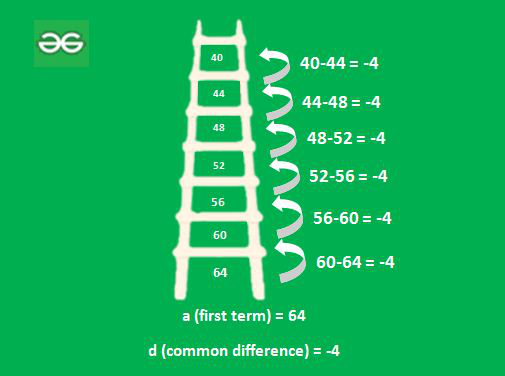Related Articles

# Evaluate the given sum: ∑k=130 (4k2-100k)

• Difficulty Level : Basic
• Last Updated : 21 Sep, 2021

Look around, from peeping at clocks or alarms in the morning to all the doings people are surrounded by numbers. The way of representing these numbers on the number line using a set of rules and symbols is termed a Number System. In the real world, generally, numbers are used ranging from 0-9, this system of numbers is called the Decimal Number System. The total number of digits present in any Number System is called the base or radix of that particular number system. In Decimal Number System, it is “10”.

Besides Decimal Number System, there is the Binary Number System (base 2 and 0, 1 as digits). The Octal Number System (base 8 and 0-7 as its digits) and the Hexadecimal Number System [base 16 and consists of digits(0-9) and six letters (A, B, C, D, E, F)]. Give it a try, add up to 5. Seems easy, doesn’t it? Possibly it might have been simply calculated (1 + 2 + 3 + 4 + 5) in your head. But what if asked to add the first 1000 Natural numbers? Stuck? Don’t be, in Mathematics, some properties are used and formulas for the same.

Attention reader! All those who say programming isn't for kids, just haven't met the right mentors yet. Join the  Demo Class for First Step to Coding Coursespecifically designed for students of class 8 to 12.

The students will get to learn more about the world of programming in these free classes which will definitely help them in making a wise career choice in the future.

### Arithmetic Progression (AP)

It is the sequence of terms having the same difference between the consecutive terms. For example, consider a ladder, its rungs are in descending order i.e. biggest at the bottom and smallest at the top. As shown, the lengths of rungs are (64, 60, 56, 52, 48, 44, 40) cm starting from the bottom.It can be seen that, there’s a common difference of -4 in all the terms therefore, it’s an AP. Now,

a2 = a + (1)(d) = 64 + (1)(-4)

a3 = a + 2d = 64 + (2)(-4) = 56

Similarly, an (nth term) = a + (n-1)d

Sum of a series is given by, Sn = a + a2 + a3 +…+ a

= a + (a + d) + (a + 2d) +…+ (a + (n-1)d)By using AP, properties of sum are obtained,

Sum of first n natural numbers = 1+2+3+…+n = ∑ n

In general terms summation is represented by

Here is read as Sigma and it means summation.

It is an AP with

a (first term) = 1

d (common difference) = 1Similarly, by using Binomial Expansion following results can be achieved

Sum of squares of first n Natural numbers(N)Sum of cubes of first n Natural numbers(N)### Evaluate the given sum:Solution:=> 37820 – 46500

=> -8680

Step 1: In the first step,property of addition where u is the upper limit and l represents the lower limit is used as :Step 2: Constant values 4 and 100 are taken out as common respectively becauseStep 3: In the 3rd step, results of sum of first n and squares of first n Natural Numbers are used and the value of k is put 30 because summation is ranging from 1-30 and then it is simply solved.

### Similar Problems

Question 1: Use the properties and formulas to evaluate the given sumSolution:=> 16562 – 2457 – 299

=> 13806

Question 2: Use the properties and formulas to evaluate the given sum,Solution:=> -4356 + 3542 + 1782 + 330

=> 1298

My Personal Notes arrow_drop_up